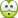# What is the angle of incidence?

## Homework Statement

A light ray shines from a diamond (n = 2.42) into water (n = 1.33), and no refraction occurs. What is the angle of incidence?

1. 30°

2. 45°

3. 60°

sin θc = nr/ni ?

## The Attempt at a Solution

I thought that by finding the critical angle then anything above that would be the angle of incidence where total internal reflection occurs therefore no refraction? But that would leave two right answers which cannot be the case. Is it actually just zero, and I am thinking about it wrong?

θi = sin-1 (1.33 sin 90°)/ 2.42 = 33.3 degrees

haruspex
Homework Helper
Gold Member
I searched for definitions of refraction. Many say it is a change in direction of the light caused by the change in medium. That would make reflection an example of refraction. Others word it as the bending of the light ray. Yet others say both, as though those descriptions are equivalent.
I would go with the first since that makes the question answerable.

I think it's a critical angle problem (limit angle) like you've done. I think your calculations are ok. , then solution is number 2.

haruspex
Homework Helper
Gold Member
I think it's a critical angle problem (limit angle) like you've done. I think your calculations are ok. , then solution is number 2.
Perhaps, but there are several reasons to think otherwise.
If you count reflection as a type of refraction, and it seems to me that at least some authorities do, then the only correct answer is 1 (0°). 30 is not close enough to 33.3 in my view.
If you do not count reflection as refraction then 30° is the only incorrect answer!

Perhaps, but there are several reasons to think otherwise.
If you count reflection as a type of refraction, and it seems to me that at least some authorities do, then the only correct answer is 1 (0°). 30 is not close enough to 33.3 in my view.
If you do not count reflection as refraction then 30° is the only incorrect answer!
Yes of course!!!!
I forgot the trivial solution theta=0. Also my English is not native and then I did not understand your previous message well.

Reflection and refraction aren't excluding phenomena, but in the trivial case theta=0 and beyond the critical (or limit) angle refraction do not appears.
Please correct me if there are some mistake in this sentence.kuruman
Homework Helper
Gold Member
2021 Award
If you count reflection as a type of refraction, and it seems to me that at least some authorities do, then the only correct answer is 1 (0°).
I don't get it. The question states that "no refraction occurs". In this case, as given by the Fresnel equation, the reflection coefficient is ##R=\left | \frac{n_2-n_1}{n_2+n_1} \right |^2=8.5\%##. This means that 91.5% is transmitted. Doesn't the transmitted light count as "refracted"? It seems to me that no refraction would occur if the angle of incidence exceeded the critical angle of 33.3o. I don't think this is a well-crafted question.

ehild
Homework Helper

## Homework Statement

A light ray shines from a diamond (n = 2.42) into water (n = 1.33), and no refraction occurs. What is the angle of incidence?

1. 30°

2. 45°

3. 60°
Is it refraction or refracted light ?
Wiki says that Refraction is the change in direction of wave propagation due to a change in its transmission medium.
At zero angle of incidence the light beam does not change direction, you can take that no refraction occurs.
Refraction means bending of the light ray in the new medium, because of the different speed of propagation. Above the critical angle, the light does not enter into the medium with lower refractive index, so there is no refracted light,

The problems and questions of physics exams should be written with the same accuracy with which the laws of physics are written.